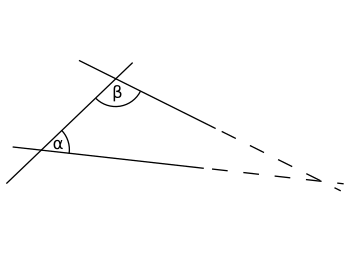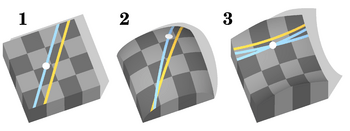# Parallel postulate facts for kids

Kids Encyclopedia FactsIf the sum of the inner angles α (alpha) and β (beta) is less than 180°, the two lines will intersect somewhere, if both are prolonged to infinity.

In geometry the parallel postulate is one of the axioms of Euclidean geometry. Sometimes it is also called Euclid's fifth postulate, because it is the fifth postulate in Euclid's Elements.

The postulate says that:

if you cut a line segment with two lines, and the two interior angles the lines form add up to less than two right angles, then the two lines will eventually meet if you extend them long enough.

The field of geometry which follows all of Euclid's axioms is called Euclidean geometry. Geometries which do not follow all of Euclid's axioms are called non-Euclidean geometry.

## History

Some mathematicians thought that Euclid's fifth postulate was much longer and more complicated than the other four postulates. Many of them thought that it could be proven from the other simpler axioms. Some mathematicians announced they had proven the proposition from the simpler propositions, but they all turned out to be mistaken. Euclid is also known as Euclid of Alexandria.

### Playfair's axiom

Another more recent proposition known as Playfair's axiom is similar to Euclid's fifth postulate. It says that:

Given a straight line and a point not on this line, you can only draw one straight line through this point that will not meet the other straight line.

In fact, mathematicians found out that this axiom is not only similar to Euclid's fifth postulate, but has exactly the same implications. Mathematically, the two propositions are called 'equivalent' propositions. Today Playfair's axiom is used more often by mathematicians than Euclid's original parallel postulate.

### Non-Euclidean geometryEuclidean, elliptical and hyperbolic geometry. The Parallel Postulate is satisfied only for models of Euclidean geometry.

Eventually some mathematicians tried to build new geometries without using the axiom. One of these new geometries is now called elliptic geometry. In elliptic geometry the parallel postulate is replaced by an axiom that states that:

Given a straight line and a point not on this line, you cannot draw a straight line through this point that will not eventually cross the other straight line.

Mathematicians found that when they replaced Euclid's fifth postulate with this axiom, they were still able to prove many of Euclid's other theorems. This formed a new geometry called elliptic geometry.

One way to imagine elliptic geometry is by thinking of the surface of a globe. On a globe, lines of longitude appear to be parallel at the equator, but they all meet at the poles.

Elliptic geometry was shown to be consistent. This proved that Euclid's fifth postulate was independent of the other postulates. After this, mathematicians mostly stopped trying to prove the fifth postulate from the other four postulates. Instead, many mathematicians began studying other geometries that do not follow Euclid's fifth postulate.

Another axiom mathematicians sometimes replace Euclid's fifth axiom with says that:

Given a straight line and a point not on this line, you can draw at least two straight lines through this point that will not eventually cross the other straight line.

This is called hyperbolic geometry.

Another geometry simply removes Euclid's fifth postulate and does not replace it with anything. This is called neutral geometry or absolute geometry.Parallel postulate Facts for Kids. Kiddle Encyclopedia.